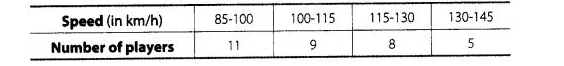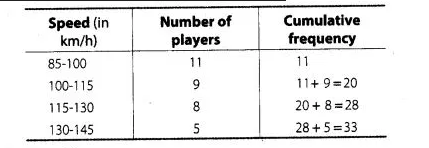# The maximum bowling speeds,

Question:

The maximum bowling speeds, in km per hour, of 33 players at a cricket coaching centre are given as followsCaluculate the median bowling speed.

Solution:

First we construct the cumulative frequency tableIt is given that, $n=33$

$\therefore$ $\frac{n}{2}=\frac{33}{2}=16.5$

So, the median class is $100-115$.

where, $\quad$ lower limit $(l)=100$,

frequency $(t)=9$,

cumulative frequency $(c f)=11$

and $\quad$ class width $(h)=15$

$\therefore$ Median $=l+\frac{\left(\frac{n}{2}-c f\right)}{f} \times h$

$=100+\frac{(16.5-11)}{9} \times 15$

$=100+\frac{5.5 \times 15}{9}=100+\frac{82.5}{9}=100+9.17$

$=109.17$

Hence, the median bowling speed is 109.17 km/h.